# 07-401/Andrei Litvin Poly Solution

Jump to: navigation, search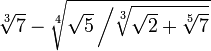$\sqrt{7} - \sqrt{\sqrt{5}\left/\sqrt{\sqrt{2}+\sqrt{7}}\right.}$ See the "Just for Fun" part of 07-401/Homework Assignment 7

Here is what I have so far in terms of polynomials:

Polynomial with root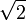$\sqrt{2}$: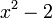${x}^{2} - 2$ (duh)

Polynomial with root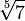$\sqrt{7}$: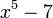$x^5 - 7$ (duh as well)

Polynomial with root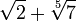$\sqrt{2} + \sqrt{7}$: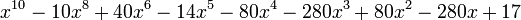$x^{10} - 10x^8 + 40x^6 - 14x^5 - 80x^4 - 280x^3 + 80x^2 - 280x + 17$ (more interesting!)

Polynomial with root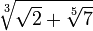$\sqrt{\sqrt{2} + \sqrt{7}}$: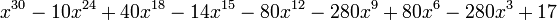$x^{30} - 10x^{24} + 40x^{18} - 14x^{15} - 80x^{12} - 280x^9 + 80x^6 - 280x^3 + 17$ (similar to the above)

Polynomial with root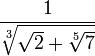${1} \over {\sqrt{\sqrt{2} + \sqrt{7}}}$: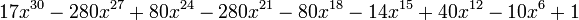$17x^{30} - 280x^{27} + 80x^{24} - 280x^{21} - 80x^{18} - 14x^{15} + 40x^{12} - 10x^6 + 1$
(just invert the above .. quite the same as the above 2)

Polynomial with root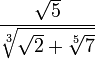${\sqrt{5}} \over {\sqrt{\sqrt{2} + \sqrt{7}}}$: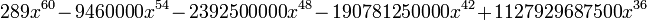$289x^{60} - 9460000x^{54} - 2392500000x^{48} - 190781250000x^{42} + 1127929687500x^{36}$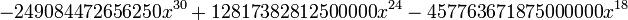$- 249084472656250x^{30} + 12817382812500000x^{24} - 457763671875000000x^{18}$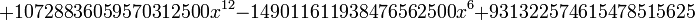$+ 10728836059570312500x^{12} - 149011611938476562500x^6 + 931322574615478515625$
(ok .. this is ugly)

Polynomial with root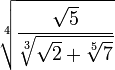$\sqrt{{\sqrt{5}} \over {\sqrt{\sqrt{2} + \sqrt{7}}}}$: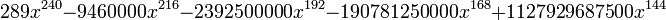$289x^{240} - 9460000x^{216} - 2392500000x^{192} - 190781250000x^{168} + 1127929687500x^{144}$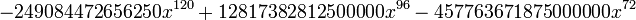$- 249084472656250x^{120} + 12817382812500000x^{96} - 457763671875000000x^{72}$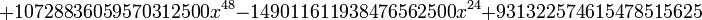$+ 10728836059570312500x^{48} - 149011611938476562500x^{24} + 931322574615478515625$
(very ugly .. especially considering you are not done yet and the next matrix to compute will be quite large)

This is how far I got so far. The program itself will do any polynomials just fine (I tweaked it a lot to get the final poly, so it may still have some bugs, however it works). The problem is working with such large numbers. The reducing part will multiply with 289 each time a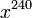$x^{240}$ is reduced, so we could potentially get coefficients of the order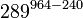$289^{964 - 240}$, which is very large indeed. On my computer, I get about 30% done, when it starts running out of memory and I had to stop. This is why the program just generates the matrices for the last part (and by the way, Maxima crashed when trying to load the matrix to invert, so I will have to find another program that will handle such large sets of data)

Comments by Dror. Great work! Your grade for the class is bounded below by 80; the exams and HW will determine which fraction of the other 20 you will get. To raise your lower bound to 85, you'll have to post the programs (either here, as text files, or elsewhere with a link from here) along with a brief manual page that will allow anybody else to run them. To raise your lower bound to 90, you'll also have to tell me, I don't care how, what is the second largest coefficient that occurs in the solution to the overall problem. The largest is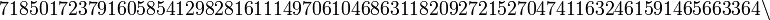$718501723791605854129828161114970610468631182092721527047411632461591465663364\backslash$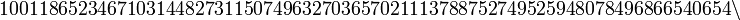$100118652346710314482731150749632703657021113788752749525948078496866540654\backslash$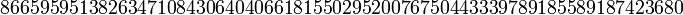$8665959513826347108430640406618155029520076750443339789185589187423680$

(223 decimal digits)

## Program

A zip file containing the program can be downloaded here (I hope this works .. if not send me an e-mail).

It does require Python (I developed and tested it with version 2.5) and the file you probably want to edit is "test.py" (or you can also create your own program files if you wish).

It starts with a statement of the form "x = Poly()" which by default creates the polynomial "x". The object overloads +,-,* and ** (power),so that you can build your own fancy polynomials (e.g. "x**2 + 4*x + 1" or "(x + 256)*((x**34 + 1)**3) + 1).

After you have built one or more polynomials (the sample starts with simple ones, like "x - 7" and " x- 5), you have an array of functions that you can build new polynomials. These are:

• "solve_inverse(p)":
• if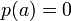$p(a) = 0$ then return q, such that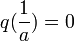$q({1 \over a}) = 0$
• "solve_sqrt(p, n)":
• if$p(a) = 0$, then return q, such that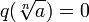$q(\sqrt[n]{a}) = 0$
• "solve_sum(p1, p2)"
• if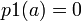$p1(a) = 0$ and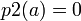$p2(a) = 0$, then return q, such that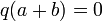$q(a+b) = 0$
• "solve_diff(p1, p2)"
• if$p1(a) = 0$ and$p2(a) = 0$, then return q, such that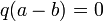$q(a-b) = 0$
• "solve_prod(p1, p2)"
• if$p1(a) = 0$ and$p2(a) = 0$, then return q, such that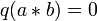$q(a*b) = 0$

Now you are ready to do things like:

 from poly import *        # these imports are needed
from polysolve import *

x = Poly()                # the most basic poly you can get

p1 = solve_sum(x**3 - 3, x**5 - 6)
p2 = solve_sum(x**2 - 2, x - 1)
p3 = solve_sum(p1, p2)

print p3